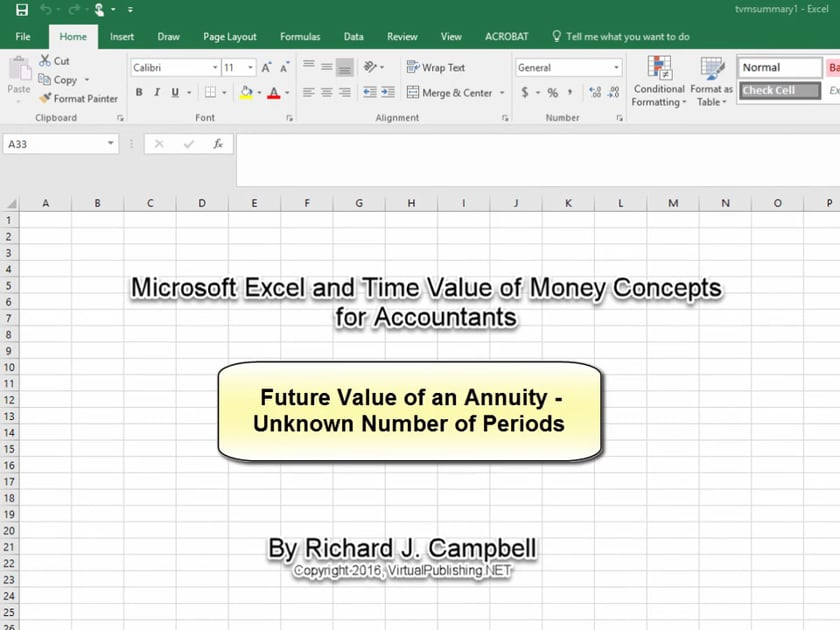## TVM12 - Future Value of an Annuity, Unknown Number of Periods## Audio Transcript

This is a future value of an annuity problem in which the number of periods is the unknown.

Suppose that a company’s goal is to accumulate \$145,100 by making periodic deposits of \$25,000 at the end of each year, which will earn 7.5% compounded annually while accumulating. How many deposits must it make? The \$145,100 represents the future value of how many \$25,000 deposits at a 7.5% annual rate of interest?

This is a future value of an annuity problem, in which the number of periods is the unknown.

First, plug in the known values into the appropriate cells:

The Payment – PMT – is (\$25,000)

The interest rate – Rate – is 7.5%

The future value – FV  – is \$145,100.00

The function wizard pops up

Select Rate,

Select PMT,

Select NPER

Going to the formulas tab, look for the NPER function.

The function wizard pops up

Select Rate,

Select FV,

Select PMT

Hit OK

The NPER  is 5.00

Scroll to Top